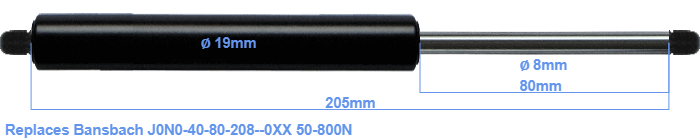# Replacement for Bansbach J0N0-40-80-208–0XX 50-800N

\$27.84

Replacement gas spring for the Bansbach J0N0-40-80-208--0XX 50-800 Newton. Thread: M8. Brand: Stabilus Industry Line.
 Force Choose an option50 Newton60 Newton80 Newton100 Newton120 Newton140 Newton150 Newton160 Newton180 Newton200 Newton220 Newton240 Newton250 Newton260 Newton280 Newton300 Newton320 Newton340 Newton350 Newton360 Newton380 Newton400 Newton420 Newton440 Newton450 Newton460 Newton480 Newton500 Newton520 Newton540 Newton550 Newton560 Newton580 Newton600 Newton620 Newton640 Newton650 Newton660 Newton680 Newton700 Newton720 Newton740 Newton750 Newton760 Newton780 Newton800 NewtonClearThe diameter of the cylinder of this gas spring is 19 mm. The diameter of the rod totals 8 mm. The rod (the chrome part) is 80 mm long. The total length of this replacement is exactly 205 mm. Note: this is the total length between the rotation points of the two mounting parts. Without the mounting parts this gas spring is 205 mm long (thread to thread). The force of this replacement is 50-800 Newton. This gas spring can replace a Bansbach gas spring, but is not an orignal Bansbach one. This replacement gas spring is a Stabilus Industry Line gas spring. Nevertheless, this gas spring can be used as replacement gas spring, because the dimensions and forces are equal.